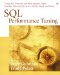# Three-Way Joins and Beyond

When more than two tables are joined, each join is scheduled and processed separatelythere is no such thing as a nested-loop within a nested-loop , even though that might seem to be the obvious strategy. Partly that's because some joins can be processed in parallel (for example, Oracle does in-parallel joining), and partly it's because a nested-loop within a nested-loop would exacerbate the problem that simple joins have (that is, that the innermost table wouldn't stay in cache). Consider this SQL statement:

` SELECT * FROM Table1, Table2, Table3    WHERE Table1.column1 = Table2.column1      AND Table2.column1 = Table3.column1 `

For a three-way join of Table1 , Table2 , and Table3 , the strategy is to merge Table1 and Table2 to a single ( Table1_2 ) table, and then merge ( Table1_2 ) with Table3 . The questionWhich expression is evaluated first?is answered in the usual way: The expression with the most points goes first (see Chapter 2, "Simple Searches"). After that, though, some shuffling occurs that depends on the closeness of the second expression to be evaluated to the first expressionso the expression with the Chapter 2, "Simple Searches"but keep in mind that, when joins are involved, those rules are no more than a weak hint.

You can expect the DBMS optimizer to start going wrong if five or more joins are in the query (until recently Microsoft's admitted limit was four). You can get around such restrictions by making temporary tables from five-table joins and then joining the temporary tables, but the fact is that your guess will be no better than that of your DBMS vendor. For a multiway join, finding the shortest path is reminiscent of a traveling salesman problem and becomes more and more complex as you add tables.

For Microsoft and Sybase, there's a common recommendation: Add a redundant WHERE clause so that the optimizer will have more choices about paths. Here's an example:

` SELECT * FROM Table1, Table2, Table3    WHERE Table1.column1 = Table2.column1      AND Table2.column1 = Table3.column1      AND Table1.column1 = Table3.column1 /* the final AND is redundant but put it in anyway */ `

The thinking here is: If the optimizer is good, it will throw away the redundant clause, but if the optimizer is bad, it will benefit from the redundant clause because it now has a choice of all six possible join ordersincluding Table1 to Table3 , and Table3 to Table1 which it wouldn't otherwise notice.

When we added the redundant WHERE clause to our example, we had to choose whether to add Expression #1 or Expression #2:

` Expression #1: ... WHERE Table1.column1 = Table3.column1 Expression #2: ... WHERE Table3.column1 = Table1.column1 `

The best way to decide how to phrase a redundant WHERE clause is to figure out which table will be the outer table in your first join and base your decision on that. (You lose this choice if you use a USING or an ON clause.) Don't try to change clauses if you have a working query that contains an outer join.

## Sybase and the Total Density Statistic

A table's density usually depends on the sensitivity of the index and is one of the items that the optimizer can gather during statistics or analysis. The density of a table is calculated as the fraction of rows that can be returned for a query. For example, if a table has 1,000 rows and density is 0.01, then 10 rows might match.

The Sybase optimizer uses a "Total Density" statistic when it needs to figure out the join order. Since the true cost of a multiway join depends heavily on the size of the outer table after each stage of the join (the smaller the better), Sybase just calculates the density for the order:

` ((Table1 JOIN Table2) then (Table2 JOIN Table3)) `

and compares the result with what would be returned for the order:

` ((Table2 JOIN Table3) then (Table1 JOIN Table2)) `

Sybase won't cost-compare more than four joins at once.SQL Performance Tuning
ISBN: 0201791692
EAN: 2147483647
Year: 2005
Pages: 125

Similar book on Amazon